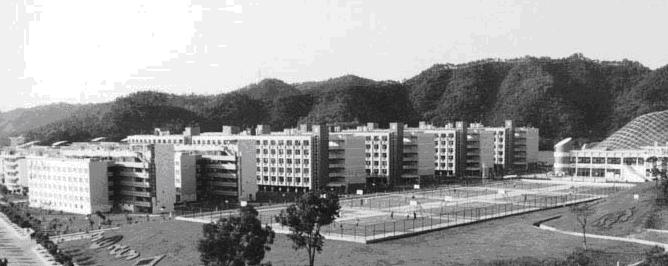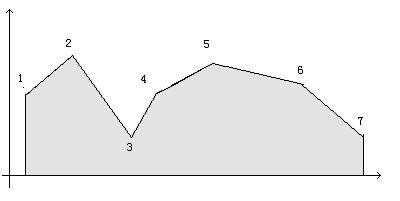20 May 2014

# 题目

#### `Problem Description`

```The 1st Annual Guangdong Collegiate Programming Contest (GDCPC) was held in Zhuhai Campus of Zhongshan(SunYat-Sen) University last year. And now we gather again for the 2nd GDCPC in the same wonderful place. After the contest, most of the participants will probably have a jaunt around the beautiful Campus.As you have seen, the main attraction of this place is the green hills surrounding. And a problem comes up now. What is the area of cross section of the hills?
This range of hills contains peaks and valleys. So before calculating the area, we have to firstly scale and write down the coordinates of the turning points. In the following graph, 7 turning points of a hill are marked.```

#### `Input`

``````The first line of the input is a positive integer T.T is the number of the test cases followed.
The first line of each test cases contains only one positive integer N(1<N<=1000) which represents the number of the turning points. Then N lines follow and the ith line contains two nonnegative integers Xi(0<=Xi<=10000),Yi(0<=Yi<=10000) which represent the coordinate of the ith turning point. It is assumed that the given turning points is in X-coordinate increasing order, i.e. Xi<Xj when i<j.
``````

#### `Output`

``````The output of the program should consist of one line of output for each test case. The output of each test case only contains one real number which represents the area of the cross section of the hills. The real numbers are rounded to one decimal digit. No redundant spaces are needed.
``````

#### `Sample Input`

``````2
2
1 4
3 4
2
2 7
3 9
``````

#### `Sample Output`

``````8.0
8.0
``````

# 解题思路

``````将图形分隔成若干个直角梯形，算出总面积就完事了。
``````